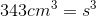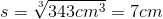## Example Questions

### Example Question #2 : Solid Geometry

The number of square units in the surface area of a cube is twice as large as the number of cubic units in its volume. What is the cube's volume, in cubic units?

108

216

9

27

36

27

Explanation:

The number of square units in the surface area of a cube is given by the formula 6s2, where s is the length of the side of the cube in units. Moreover, the number of cubic units in a cube's volume is equal to s3

Since the number of square units in the surface area is twice as large as the cubic units of the volume, we can write the following equation to solve for s:

6s2 = 2s3

Subtract 6s2 from both sides.

2s3 – 6s2 = 0

Factor out 2s2 from both terms.

2s2(s – 3) = 0

We must set each factor equal to zero.

2s2 = 0, only if s = 0; however, no cube has a side length of zero, so s can't be zero.

Set the other factor, s – 3, equal to zero.

s – 3 = 0

s = 3

This means that the side length of the cube is 3 units. The volume, which we previously stated was equal to s3, must then be 33, or 27 cubic units.

### Example Question #3 : Solid Geometry

You own a Rubik's cube with a volume of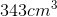. What is the edge length of the cube?

No enough information to solve.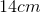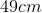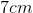Explanation:

You own a Rubik's cube with a volume of. What is the edge length of the cube?

To solve for edge length, think of the volume of a cube formula: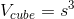Now, we have the volume, so just rearrange it to solve for side length: